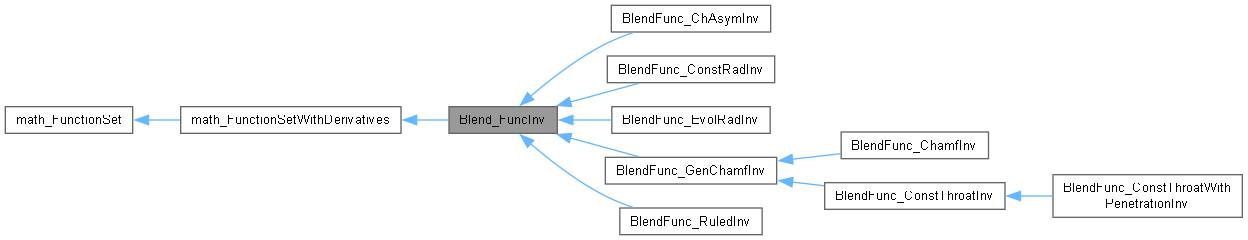Open CASCADE Technology  7.7.0.dev

# Blend_FuncInv Class Referenceabstract

Deferred class for a function used to compute a blending surface between two surfaces, using a guide line. This function is used to find a solution on a restriction of one of the surface. The vector <X> used in Value, Values and Derivatives methods has to be the vector of the parametric coordinates t,w,U,V where t is the parameter on the curve on surface, w is the parameter on the guide line, U,V are the parametric coordinates of a point on the partner surface. More...

`#include <Blend_FuncInv.hxx>`

Inheritance diagram for Blend_FuncInv:[legend]

## Public Member Functions

Standard_Integer NbVariables () const
Returns 4. More...

virtual Standard_Integer NbEquations () const =0
returns the number of equations of the function. More...

virtual Standard_Boolean Value (const math_Vector &X, math_Vector &F)=0
computes the values <F> of the Functions for the variable <X>. Returns True if the computation was done successfully, False otherwise. More...

virtual Standard_Boolean Derivatives (const math_Vector &X, math_Matrix &D)=0
returns the values <D> of the derivatives for the variable <X>. Returns True if the computation was done successfully, False otherwise. More...

virtual Standard_Boolean Values (const math_Vector &X, math_Vector &F, math_Matrix &D)=0
returns the values <F> of the functions and the derivatives <D> for the variable <X>. Returns True if the computation was done successfully, False otherwise. More...

virtual void Set (const Standard_Boolean OnFirst, const Handle< Adaptor2d_Curve2d > &COnSurf)=0
Sets the CurveOnSurface on which a solution has to be found. If <OnFirst> is set to Standard_True, the curve will be on the first surface, otherwise the curve is on the second one. More...

virtual void GetTolerance (math_Vector &Tolerance, const Standard_Real Tol) const =0
Returns in the vector Tolerance the parametric tolerance for each of the 4 variables; Tol is the tolerance used in 3d space. More...

virtual void GetBounds (math_Vector &InfBound, math_Vector &SupBound) const =0
Returns in the vector InfBound the lowest values allowed for each of the 4 variables. Returns in the vector SupBound the greatest values allowed for each of the 4 variables. More...

virtual Standard_Boolean IsSolution (const math_Vector &Sol, const Standard_Real Tol)=0
Returns Standard_True if Sol is a zero of the function. Tol is the tolerance used in 3d space. More...Public Member Functions inherited from math_FunctionSet
virtual Standard_Integer GetStateNumber ()
Returns the state of the function corresponding to the latestcall of any methods associated with the function. This function is called by each of the algorithms described later which define the function Integer Algorithm::StateNumber(). The algorithm has the responsibility to call this function when it has found a solution (i.e. a root or a minimum) and has to maintain the association between the solution found and this StateNumber. Byu default, this method returns 0 (which means for the algorithm: no state has been saved). It is the responsibility of the programmer to decide if he needs to save the current state of the function and to return an Integer that allows retrieval of the state. More...

virtual ~math_FunctionSet ()

## Detailed Description

Deferred class for a function used to compute a blending surface between two surfaces, using a guide line. This function is used to find a solution on a restriction of one of the surface. The vector <X> used in Value, Values and Derivatives methods has to be the vector of the parametric coordinates t,w,U,V where t is the parameter on the curve on surface, w is the parameter on the guide line, U,V are the parametric coordinates of a point on the partner surface.

## ◆ Derivatives()

 virtual Standard_Boolean Blend_FuncInv::Derivatives ( const math_Vector & X, math_Matrix & D )
pure virtual

returns the values <D> of the derivatives for the variable <X>. Returns True if the computation was done successfully, False otherwise.

Implements math_FunctionSetWithDerivatives.

## ◆ GetBounds()

 virtual void Blend_FuncInv::GetBounds ( math_Vector & InfBound, math_Vector & SupBound ) const
pure virtual

Returns in the vector InfBound the lowest values allowed for each of the 4 variables. Returns in the vector SupBound the greatest values allowed for each of the 4 variables.

## ◆ GetTolerance()

 virtual void Blend_FuncInv::GetTolerance ( math_Vector & Tolerance, const Standard_Real Tol ) const
pure virtual

Returns in the vector Tolerance the parametric tolerance for each of the 4 variables; Tol is the tolerance used in 3d space.

## ◆ IsSolution()

 virtual Standard_Boolean Blend_FuncInv::IsSolution ( const math_Vector & Sol, const Standard_Real Tol )
pure virtual

Returns Standard_True if Sol is a zero of the function. Tol is the tolerance used in 3d space.

## ◆ NbEquations()

 virtual Standard_Integer Blend_FuncInv::NbEquations ( ) const
pure virtual

returns the number of equations of the function.

Implements math_FunctionSetWithDerivatives.

## ◆ NbVariables()

 Standard_Integer Blend_FuncInv::NbVariables ( ) const
virtual

Returns 4.

Implements math_FunctionSetWithDerivatives.

## ◆ Set()

 virtual void Blend_FuncInv::Set ( const Standard_Boolean OnFirst, const Handle< Adaptor2d_Curve2d > & COnSurf )
pure virtual

Sets the CurveOnSurface on which a solution has to be found. If <OnFirst> is set to Standard_True, the curve will be on the first surface, otherwise the curve is on the second one.

## ◆ Value()

 virtual Standard_Boolean Blend_FuncInv::Value ( const math_Vector & X, math_Vector & F )
pure virtual

computes the values <F> of the Functions for the variable <X>. Returns True if the computation was done successfully, False otherwise.

Implements math_FunctionSetWithDerivatives.

## ◆ Values()

 virtual Standard_Boolean Blend_FuncInv::Values ( const math_Vector & X, math_Vector & F, math_Matrix & D )
pure virtual

returns the values <F> of the functions and the derivatives <D> for the variable <X>. Returns True if the computation was done successfully, False otherwise.

Implements math_FunctionSetWithDerivatives.

The documentation for this class was generated from the following file: